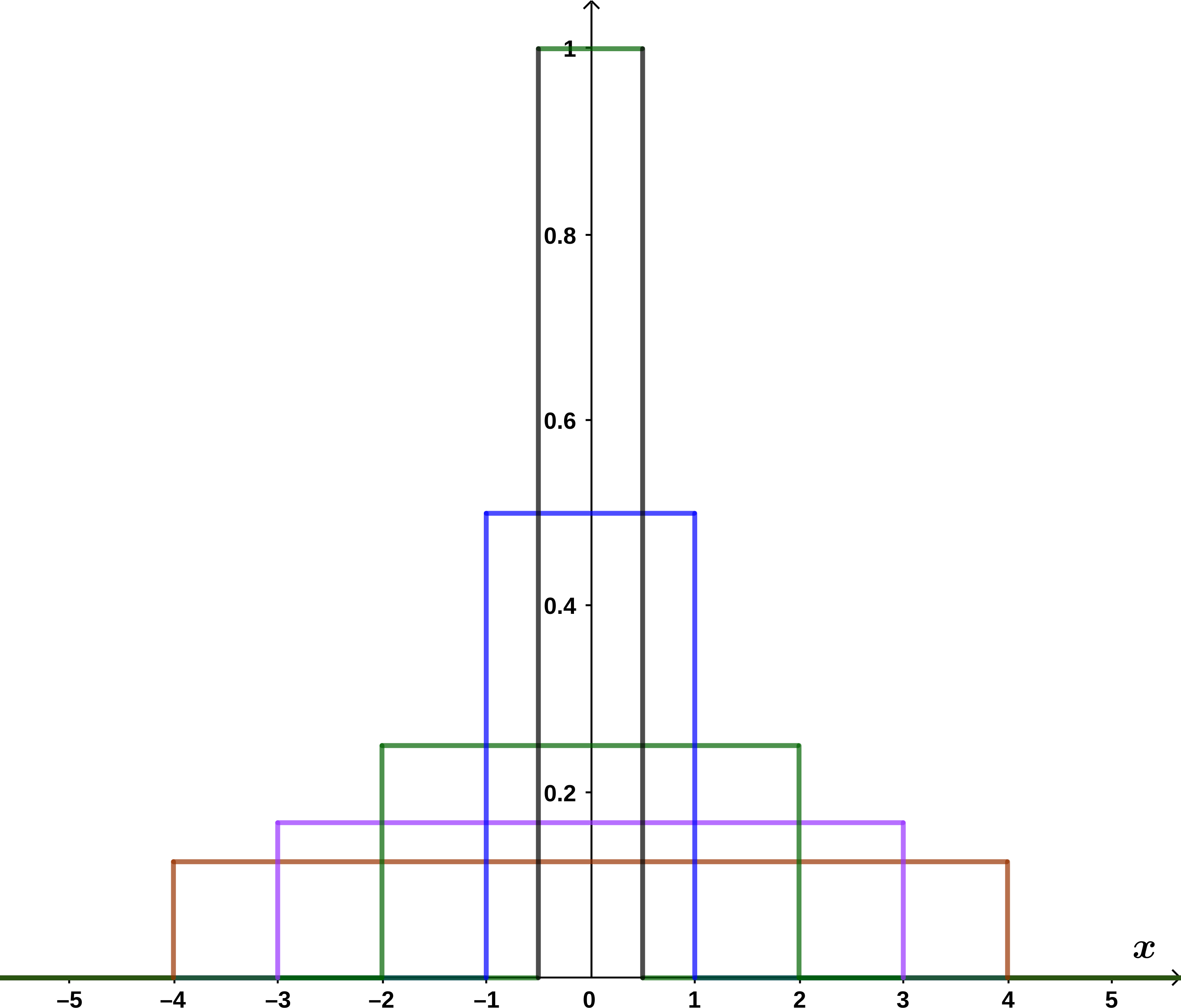## Section11.2The Dirac Delta Function

The Dirac delta function $$\delta(x)$$ is not really a “function”. It is a mathematical entity called a distribution which is well defined only when it appears under an integral sign. It has the following defining properties:

\begin{equation} \delta(x) = \cases{0, \qquad \amp if $x\not= 0$\cr \infty, \qquad \amp if $x=0$\cr}\tag{11.2.1} \end{equation}
\begin{equation} x\,\delta(x) \equiv 0\tag{11.2.3} \end{equation}

It may be easiest to think of the delta function as the limit of a sequence of steps, each of which is higher and narrower than the previous step, such that the area under the step is always one; see Figure Figure 11.2.1.Figure 11.2.1. The function $$\delta(x)$$ can be approximated by a series of steps that get progressively thinner and higher in such a way that the area under the curve is always equal to one.

The properties of the delta function allow us to compute

\begin{align*} \int_{-\infty}^{\infty} f(x)\,\delta(x) \,dx \amp = \int_{-\infty}^{\infty} f(0)\,\delta(x) \,dx\\ \amp = f(0) \int_{-\infty}^{\infty} \delta(x) \,dx \nonumber\\ \amp = f(0) \nonumber \end{align*}

We can shift the “spike” in the delta function as usual, obtaining $$\delta(x-a)\text{.}$$ This shifted delta function satisfies

\begin{equation} \int_{-\infty}^{\infty} f(x)\,\delta(x-a) \,dx = f(a)\tag{11.2.4} \end{equation}

Thus, the Dirac delta function can be used to pick out the value of a function at any desired point.

We can relate the delta function to the step function in the following way. Consider the function $$g(x)$$ given by the integral

\begin{equation} g(x)=\int_{-\infty}^x \delta(u-a)\,du\tag{11.2.5} \end{equation}

Notice the variable $$x$$ in the upper limit of the integral. The value of this function $$g(x)$$ is $$0$$ if we stop integrating before we reach the peak of the delta function, i.e. for $$x\lt a\text{.}$$ If we integrate through the peak, the value of the integral is $$1\text{,}$$ i.e. for $$x>a\text{.}$$ Thus, we have argued that the value of the integral, thought of as a function of $$x\text{,}$$ is just the step function

\begin{equation} \Theta(x-a) = \int_{-\infty}^x \delta(u-a)\,du\tag{11.2.6} \end{equation}

(Recall that we don't really care about the choice of $$\Theta(0)\text{,}$$ so we don't need to worry about the value of this function if we stop integrating exactly at $$x=a\text{.}$$)

If the step function is the integral of the delta function, then the delta function must be the derivative of the step function.

\begin{equation} \frac{d}{dx}\Theta(x-a) = \delta(x-a)\tag{11.2.7} \end{equation}

You should be able to persuade yourself that this statement is reasonable geometrically if you think of the derivative of a function as representing its slope.

Prove that the derivative of the step function is the delta function, i.e. prove Equation (11.2.7).

Solution.

We need to show that

\begin{equation} \Int_{b}^{c} f(x)\,\delta(x-a) \,dx = f(a)\tag{11.2.8} \end{equation}

for $$b\lt a\lt c\text{,}$$ where

\begin{equation} \delta(x-a)=\frac{d}{dx}\,\Theta(x-a)\tag{11.2.9} \end{equation}

The main strategy is to use integration by parts, paying strict attention to the limits of integration.

\begin{align*} \Int_{b}^{c} f(x)\,\delta(x-a) \,dx \amp = \Int_{b}^{c} f(x)\,\frac{d}{dx}\, \Theta(x-a) \,dx \nonumber\\ \amp = \left. f(x)\, \Theta(x-a)\right|_b^c - \Int_{b}^{c} \frac{d}{dx}(f(x))\,\Theta(x-a) \,dx \nonumber\\ \amp = \left\{ f( c )-0\right\} - \Int_{a}^{c} \frac{d}{dx}(f(x))\,dx \nonumber\\ \amp = f( c )-\left\{\left. f(x)\right|_a^c\right\} \nonumber\\ \amp = f( c )-\left\{f( c )-f(a)\right\} \nonumber\\ \amp = f(a) \end{align*}